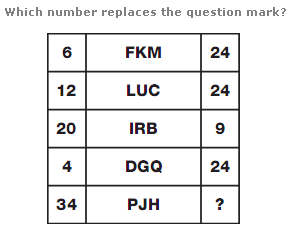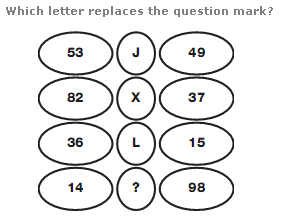# Puzzles - Logical puzzles

Exercise : Logical puzzles
28.:
M
Explanation
:
In each column, add up each number and put the letter with this sum in the bottom circle.

29.:
0
Explanation
:
In each row, the left hand number equals the total of the even valued letters in the middle box, and the right hand number equals the total of the odd valued letters in the middle box.

30.